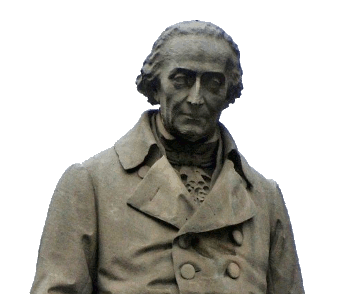### The Mass of Information

December 5, 2014

Einstein's most famous equation, at least to people who aren't that interested in general relativity, is the mass-energy equivalence equation, E = mc2. As I wrote in a previous article (Mass-Energy Equivalence, June 2, 2014), Einstein's publication of mass-energy equivalence didn't contain the E = mc2 equation; instead, Einstein wrote L = mc2, where L is the Lagrangian.

The Lagrangian (L = T - V) is the difference between the kinetic energy (T) and the potential energy (V) of a system. It's a useful concept in classical mechanics, which was essentially the only developed form of mechanics when Einstein published his paper, just five years after the discovery of the quantum of energy by Max Planck.Joseph-Louis Lagrange

The list of things named after Lagrange is huge, but he's best known in popular science for the Lagrange points, the points where an object can maintain a stable orbit with respect to the Earth and Moon.

(Detail of a statue of Lagrange in Turin, Italy, via Wikimedia Commons.)

The equation, as written in its traditional form, puts the emphasis of energy, but it's possible to invert the equation to associate a mass with a quantity of energy. If you have an energy, you can use Einstein's equation to calculate its equivalent mass. Such masses are small, since the speed of light, 300 million meters per second, squared, is the conversion factor between the SI units for energy and mass, the joule and the kilogram; viz., c2 = 9 x 1016 meters2/sec2.

Since even scientists don't have a good idea of the magnitude of some SI units as they relate to everyday life, we note that a kilowatt-hour is 3.6 million joules (3.6 x 106 joules). We can convert this amount of energy, enough to power a hair dryer for an hour, to an equivalent mass, which gives us just 4 x 10-8 grams (40 nanograms).

In 1961, at the time when computers were becoming more common, Rolf Landauer (1927-1999), a physicist working at IBM, investigated the thermodynamics of computing. In most cases, computing is an irreversible process, so computation should cause some energy to be lost to the environment. Landauer's calculation of the minimum energy lost per irreversible bit operation gives a result that appears obvious in retrospect; namely, E = kT ln(2), where k is the Boltzmann constant (1.38 x 10-23 J/K), T is the temperature, and ln() is the natural logarithm. The factor of 2 comes, of course, from the idea that a bit has two states.

All this is related to the concept of entropy S, as given by Boltzmann's equation, S = k ln(Ω), in which Ω is the number of possible system states, combined with the idea that the product of temperature and entropy, TS, gives the internal energy of an adiabatic system; that is, a system that doesn't exchange heat or mass with its surroundings. The minimum energy of a bit operation is very small. At room temperature (about 25°C), it's just 2.85 zeptojoules. For those who have trouble sorting their zeptos from their femtos and attos, a zeptojoule is 10-21 joule. This energy has actually been measured in a model memory cell comprising a colloidal particle moving between two potential wells.

The discussion above about the Landauer's limit in computation relates to the energy we see as the result of bit changes, and this includes the creation of a bit of information. We can associate a mass with such an energy, and that's an idea posed in a paper posted on arXiv earlier this year by Luis Herrera of the School of Physics, the Universidad Central de Venezuela, Caracas, Venezuela.[3-4] Essentially, he applies the mass-energy equivalence to the Landauer energy. As a result, the mass of a single bit of information at room temperature is about 3 x 10-35 grams, which is a hundred million times smaller than the mass of an electron (9.11 x 10-28 grams).

It's also possible to plug this energy into Heisenberg's uncertainty relation, as written in time and energy, ΔE Δt ≈ h/2π, where h is the Planck constant (6.626 x 10-34 joule-sec), to get a time t associated with the energy change. Inverting this time gives you the maximum frequency at which information can be changed, 105 GHz, which is comfortably higher than the several GHz of today's desktop computers.Portrait, from the German Federal Archives, of Werner Heisenberg, who was awarded the 1933 Nobel Prize in Physics.

Heisenberg's uncertainty relation is "Heisenbergsche Unschärferelation" in German.

(Via Wikimedia Commons.)### References:

Linked Keywords: Albert Einstein; equation; general relativity; mass-energy equivalence; scientific literature; publication; Lagrangian; kinetic energy; potential energy; physical system; classical mechanics; quantum; Max Planck; Joseph-Louis Lagrange; things named after Lagrange; Lagrange point; Turin, Italy; Wikimedia Commons; energy; mass; speed of light; meters per second; squared; conversion factor; International System of Units; SI units; joule; kilogram; scientist; everyday life; kilowatt-hour; hair dryer; gram; nanogram; computer; Rolf Landauer (1927-1999); physicist; IBM; thermodynamics; irreversible process; environment; Landauer's principle; adiabatic process; adiabatic system; bit; Boolean algebra; operation; room temperature; celsius; °C; zepto; femto; atto; conceptual model; computer data storage; memory cell; colloid; colloidal; particle; potential well; Landauer's limit; information; arXiv; School of Physics; Central University of Venezuela; Universidad Central de Venezuela; electron; Heisenberg's uncertainty relation; time; Planck constant; frequency; hertz; GHz; desktop computer; portrait photography; German Federal Archives; Werner Heisenberg; Nobel Prize in Physics; German language; A. Einstein, "Ist die Trägheit eines Körpers von seinem Energieinhalt abhängig?", Annalen der Physik, vol. 18, no. 13 (1905).